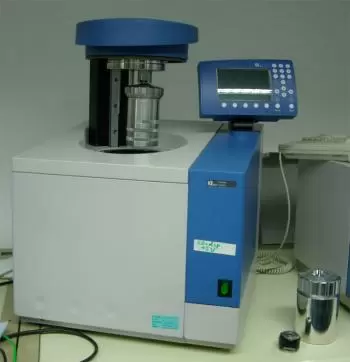# Definition of Calorimeter and Water EquivalentA calorimeter measures the amounts of heat supplied or received in any physical, chemical, or biological process. It is also used to know the specific heat of a body.

Heat transfer is not always synonymous with a temperature change. For example, during a melting phase change of water, the amount of heat is used for molecular evolution. During this process, the temperature of the water is constant (zero degrees Celsius).

If the energy changes occur at constant pressure, all the energy that enters and leaves the thermodynamic system is heat. Therefore, the calorimeter measures the amount of energy exchanged that coincides with the change in enthalpies.

An exothermic reaction is a reaction that gives off heat. Reactions that absorb heat are called endothermic reactions.

## What Is the Water Equivalent of a Calorimeter?

The water equivalent of a calorimeter is the mass of water that would behave the same as the calorimeter and lose the same heat under the same circumstances. In this way, you only have to add the number of equivalents to the water.

Calorimeters tend to include their equivalent making calculations easier.

## How Does a Calorimeter Work?

The heat capacity of an object is obtained by immersing the object in a liquid (usually water) whose initial temperature and heat capacity are known. The heat flows from the hottest element to the coldest. Since it is a closed system, all heat released is transferred to the other part.

After some time, the liquid and the object will have the same temperature and will have reached the equilibrium temperature.

By knowing these data, with the temperature difference, the specific heat of the object can be calculated from the energy conservation law.

### Calculating the Heat Exchanged

The amount of heat exchanged by an object can be determined by the following formula:

Q=m·c·Δt

Where:

• “Q” is the heat exchanged.

• “m” is the mass of the object on which we are working expressed in kg according to the S.I.

• “c” is the specific heat of the object of the experiment expressed in J/g·ºC.

• “Δt” is the temperature variation during the exchange process.

Therefore, in a calorimeter, the amount of heat that enters or leaves the object equals the heat that enters or leaves the liquid in the calorimeter. So:

mobject · cobject ·Δtobject = mliquid · cliquid · Δtliquid

## What Is the Specific Heat of Water?

The specific heat of water is 1 cal/°C·g. It means that it takes a heat of one calorie to raise the temperature of one gram of water by one degree Celsius. In the international system, they are 4186 J/g·ºC.

## Types of Calorimeters

The three most common types of calorimeters are:

• Temperature variation calorimeter: the operation is based on the change in temperature of a substance.

• Isothermal calorimeter: the operation is based on changing the state of aggregation of the substance.

• Adiabatic calorimeter: the liquid does not exchange heat with the outside environment. It is achieved by additional insulation of the apparatus with the help of an outer jacket through which the fluid circulates at the same temperature as inside. They can work in two different ways:

• First, the outer layer works at a constant temperature.

• The outer layer's temperature is mantained as close as possible to the vessel. In This case, auxiliary external heaters compensate for the heat loss.

### Differential Scanning Calorimeters

It is a technique in which the heat difference between a sample and a reference is measured as a function of temperature. The sample and reference are kept at approximately the same temperature throughout an experiment.

### Coffee Cup Calorimeters

It is a technique to get insulation when some materials are mixed. Its composition is a styrofoam cup, and sometimes it is one cup inside another. The device includes a sensitive thermometer and styrofoam.

### Bomb Calorimetry: Reaction Calorimeter

A bomb calorimeter is the same device but operates at constant volume. This type of device is used to measure the heat of combustion of a particular kind of reaction that requires high pressure.

In some chemical reactions, such as combustion, they use a heavy steel container called reaction vessel to hold the reaction. The reaction vessel is introduced into the calorimeter container, and the reaction occurs due to an external trigger.

## What Is the Composition of a Calorimeter?

The most widely used calorimeter is made of:

• A closed and completely insulated container with water.

• Device for stirring

• Thermometer.

The heat source is located inside the calorimeter container. The stirring device is used to speed up the thermodynamic change and reach thermal equilibrium sooner. Finally, the thermometer is used to know the temperature difference during the process.

Author:

Published: September 26, 2019
Last review: June 26, 2022